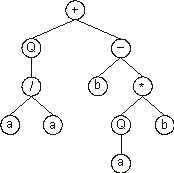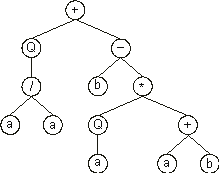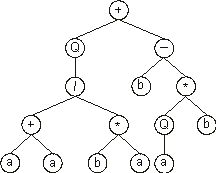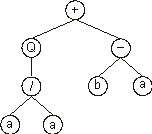GEP Book

Home
News
Author
Q&A
Tutorials
GEP Biblio
Contacts

Visit Gepsoft

 C. FERREIRA Complex Systems, 13 (2): 87-129, 2001

Gene Expression Programming: A New Adaptive Algorithm for Solving Problems

Gene Expression Programming Genes

GEP genes are composed of a head and a tail. The head contains symbols that represent both functions (elements from the function set F) and terminals (elements from the terminal set T), whereas the tail contains only terminals. Therefore two different alphabets occur at different regions within a gene. For each problem, the length of the head h is chosen, whereas the length of the tail t is a function of h and the number of arguments of the function with the most arguments n, and is evaluated by the equation:

 t = h (n-1) + 1 (3.4)

Consider a gene composed of {Q, *, /, -, +, a, b}. In this case n = 2. For instance, for h = 10 and t = 11, the length of the gene is 10 + 11 = 21. One such gene is shown below (the tail is shown in blue):

 012345678901234567890 +Q-/b*aaQbaabaabbaaab (3.5)

and it codes for the following ET:In this case, the ORF ends at position 10, whereas the gene ends at position 20.

Suppose now a mutation occurred at position 9, changing the “b” into “+”. Then the following gene is obtained:

 012345678901234567890 +Q-/b*aaQ+aabaabbaaab (3.6)

and its ET gives:In this case, the termination point shifts two positions to the right (position 12).

Suppose now that a more radical modification occurred, and the symbols at positions 6 and 7 in gene (3.5) change respectively into “+” and “*”, creating the following gene:

 012345678901234567890 +Q-/b*+*Qbaabaabbaaab (3.7)

giving the ET:In this case the termination point shifts several positions to the right (position 14).

Obviously the opposite also happens, and the ORF is shortened. For example, consider gene (3.5) and suppose a mutation occurred at position 5, changing the “*” into “a”:

 012345678901234567890 +Q-/baaaQbaabaabbaaab (3.8)

Its expression results in the following ET:In this case, the ORF ends at position 7, shortening the original ET by 3 nodes.

Despite its fixed length, each gene has the potential to code for ETs of different sizes and shapes, the simplest being composed of only one node (when the first element of a gene is a terminal) and the biggest composed of as many nodes as the length of the gene (when all the elements of the head are functions with the maximum number of arguments, n).

It is evident from the examples above, that any modification made in the genome, no matter how profound, always results in a valid ET. Obviously the structural organization of genes must be preserved, always maintaining the boundaries between head and tail and not allowing symbols from the function set on the tail. Section 5 shows how GEP operators work and how they modify the genome of GEP individuals during reproduction.

Home | Contents | Previous | Next